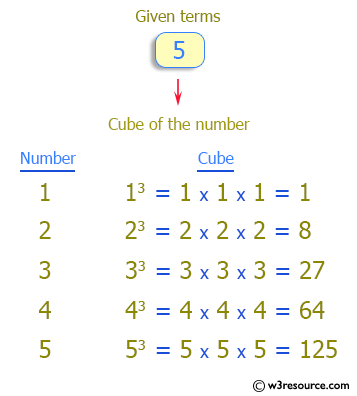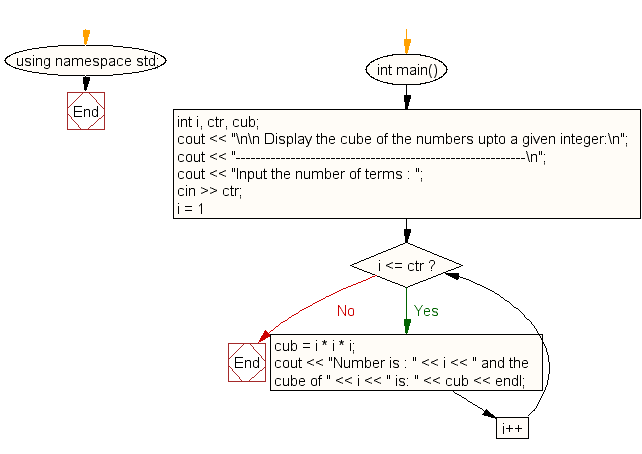﻿ C++ : Display the cube of the number upto given an integer

# C++ Exercises: Display the cube of the number upto given an integer

## C++ For Loop: Exercise-18 with Solution

Write a program in C++ to display the cube of the number up to an integer.

Visual Presentation:Sample Solution:-

C++ Code :

``````#include <iostream> // Include input-output stream header

using namespace std; // Using standard namespace to avoid writing std::

int main() // Start of the main function
{
int i, ctr, cub; // Declaration of integer variables 'i' (loop counter), 'ctr' (number of terms), 'cub' (holds the cube value)

cout << "\n\n Display the cube of the numbers upto a given integer:\n"; // Display message on the console
cout << "----------------------------------------------------------\n";
cout << "Input the number of terms : "; // Prompt the user to input the number of terms
cin >> ctr; // Read the input value as the number of terms

for (i = 1; i <= ctr; i++) // Loop from 1 to the input number of terms
{
cub = i * i * i; // Calculate the cube of the current number 'i'
cout << "Number is : " << i << " and the cube of " << i << " is: " << cub << endl; // Display the number and its cube
}
}
``````

Sample Output:

``` Display the cube of the numbers upto a given integer:
----------------------------------------------------------
Input the number of terms : 5
Number is : 1 and the cube of 1 is: 1
Number is : 2 and the cube of 2 is: 8
Number is : 3 and the cube of 3 is: 27
Number is : 4 and the cube of 4 is: 64
Number is : 5 and the cube of 5 is: 125
```

Flowchart:C++ Code Editor: